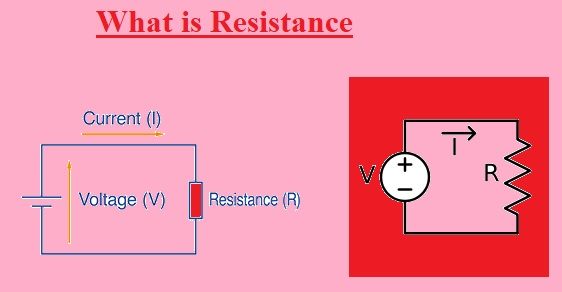Hello, readers welcome to new post. Here we will discuss Difference Between Resistance and Impedance. The basic difference between resistance and impedance is the relation of these parameters for AC and DC currents. Resistance is used to control the ac and dc flow while impedance is used for ac.

Resistance is used only for resistive circuits while impedance used for inductive resistive and capacitive loads. In this post, we will discuss different paramerts for getting the details of differences. SO let’s get started Difference Between Resistance and Impedance.

## Difference Between Resistance and Impedance

### What is Resistance

• The opposition offered in the flow of current is called resistance for the circuit. Measured in ohms and denoted with Omega.
• It is the force that opposes the flow of current. Greater the resistance less current will flow
• Resistance used in dc circuits is caused by the resistive component of circuits
• It denoted with R
• It is measured in real numbers like 10 ohms
• The frequency of dc not affect the value of resistance
• Tehre is no value of phase angle and  have magnitude
• Its formula is Resistance = Voltage / current
• Ohm law states the potential of the conductor having current flow is given as
• V=IR
• V is volts applied across the conductor
• I current flow
• R is resistance### Factors Affecting Resistance

• Tehre are some factors over resistance is dependant
• Temperature
• Material of conductive element
• COnductor length
• Cross-sectional area
• Resistance has direct relation of conductor length and inverse to A.
• R=ρL/A

## What is Impedance• The  opposition offered to the ac current due ot resistance and reactance is called impedance
• Impedance value depends on the frequency of circuits
• It has both magnitude and phase and is used for ac circuits.
• It is a complex number but has the same units as the resistance OHM.
• It is denied as Z.
• The reciprocal of impedance is admittance and its SI unit is siemens mho.
• The device used for measuring the impedance is called an impedance analyzer.
• Impedance in AC circuits comprises the effects of volts of volts induction in conductors through a magnetic field called inductance
• The electrostatic storage of shared produced through volts among the conductors is called capacitance.
• The impedance results from the inductance and capacitance called reactance.

That is all about the Difference Between Resistance and Impedance all details has been explained. If you have any issues ask in the comments. Thanks for reading have a nice day see you in next post.